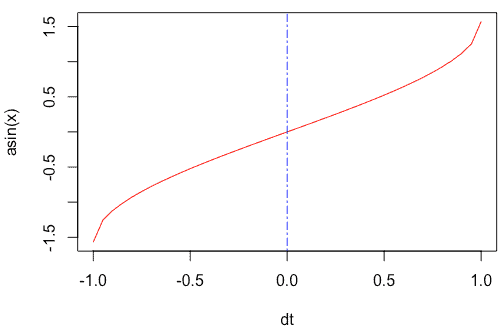# asin in R: How to Use asin() Function in R

To calculate the inverse sine value of the input numeric value in R, use the asin() function.

## asin in R

The asin() is a built-in R trigonometric function that computes the sine inverse of the given value; the returned angle is in the range -pi/2 through pi/2. The asin() method accepts vectors and returns the inverse of sine value.

### Syntax

``asin(x)``

### Parameters

The asin() function takes a column to compute on.

### Example

Define two vectors and pass them to the asin() function.

``````v1 <- -1
v2 <- 0.5

asin(v1)
asin(v2)``````

#### Output

`````` -1.570796
 0.5235988``````

If you pass the 0 to the asin() function, it will return 0.

``v3 <- 0``

#### Output

`` 0``

## Plot the asin() function to a graph

We can use the seq() function to create a series of values and pass that to the plot() function, which will create a line chart.

``````dt <- seq(-1, 1, by = 0.05)
plot(dt, asin(dt), typ = "l", col = "red")
abline(v = 0, lty = 6, col = "blue")``````

#### Output## Applying asin() function to a Vector

To define a Vector in R, use the c() function. Then pass that vector to the asin() function.

``````rv <- c(-1, 0.5, 0, 0.5, 1)
asin(rv)``````

#### Output

`` -1.5707963 0.5235988 0.0000000 0.5235988 1.5707963``

## Passing a pi to the asin() function

The pi is an inbuilt constant in R programming, and its value is 3.141593.

Let’s find the pi constant’s asin() value.

``asin(pi)``

#### Output

`````` NaN
Warning message:
In asin(pi) : NaNs produced``````

You can see that it returns NaN(not a number) in the output.

Let’s see another example of pi.

``asin(pi / 4)``

#### Output

`` 0.9033391``

That is it for this tutorial.